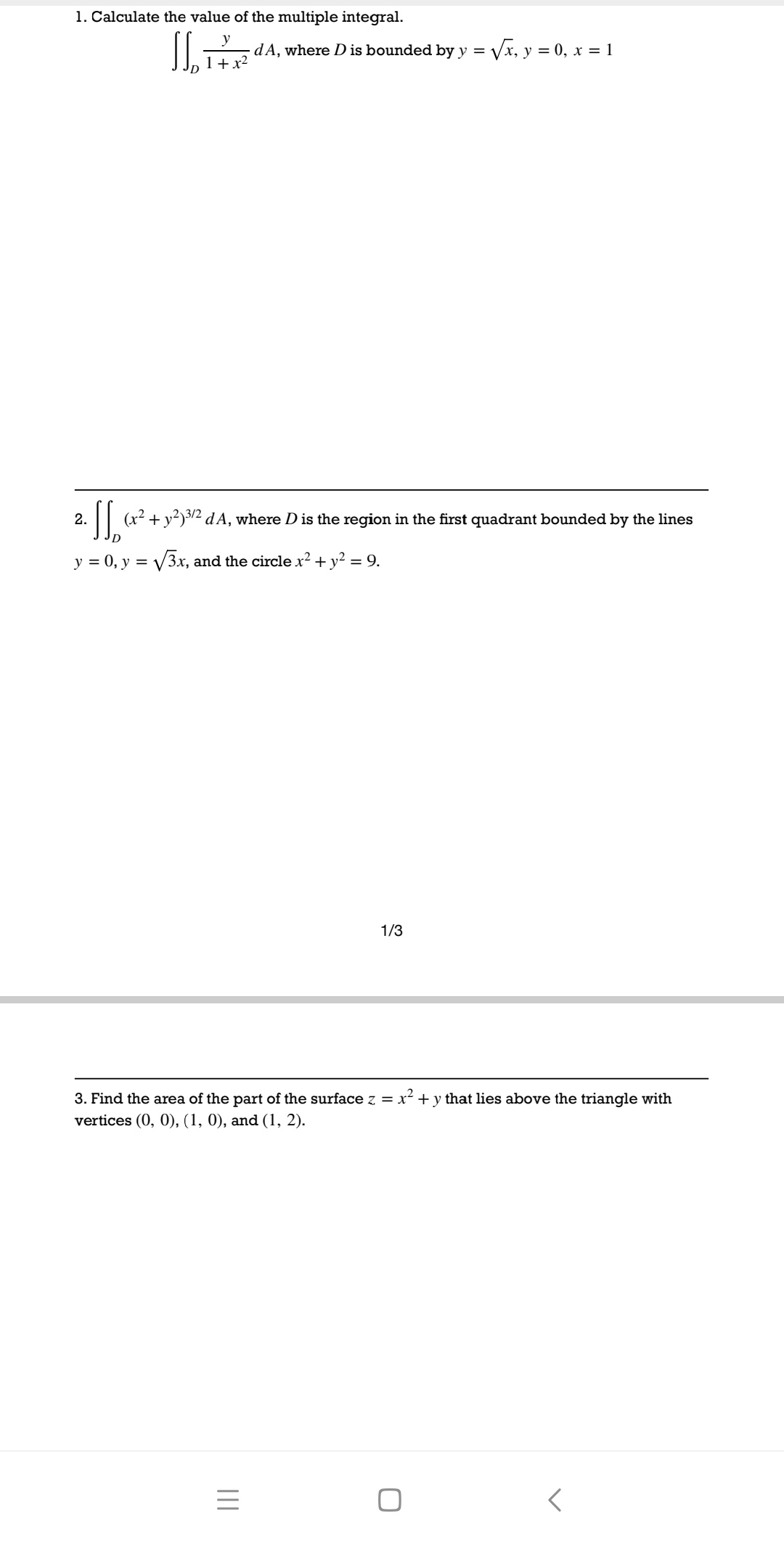### 3 questions about multiple integral1.Calculate the value of the multiple integral.dA,where D is bounded by y=√x,y=0,x=11+x2a(2+2dA,Dis he regin inth quadrant bounded by te iesy=0,y=√3x,and the circle x2+y2=9.1/33.Find the area of the part of the surface z x2+y that lies above the triangle withvertices(0,0),(1,0),and(1,2).三<